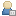###Show Posts

This section allows you to view all posts made by this member. Note that you can only see posts made in areas you currently have access to.

### Messages - Phearcia

Pages:  2 3 ... 16
1
##### Ideas / silver pieces, copper pieces, platinum pieces
« on: September 17, 2016, 09:08:38 PM »
Gold pieces are awesome, but what about other money values. I know there is the Item command. This is mainly for RPG maps, just thought it might be a good idea.

2
##### Questions and Answers / Re: How would you do this? (Vectors+HUD Shapes = Custom Waypoints)
« on: September 15, 2016, 08:05:52 PM »
Constantly updating compass, using x and z facing and 360 hudbars.

3
##### Questions and Answers / Re: making a cone shaped targeting effect
« on: September 11, 2016, 07:40:02 PM »
I think what I may do is make a script that detects the the view numbers and it's between a certain range I just increase the .3 view modifier to a .6.

Aaaand that doesn't work.

4
##### Questions and Answers / Re: How would I go about doing this?
« on: September 11, 2016, 07:18:35 PM »

5
##### Questions and Answers / Re: making a cone shaped targeting effect
« on: September 11, 2016, 07:08:46 PM »
The boomerang ordeal. I think it may actually be similar. The view x and view z vectors intersect like scissors in a way. How did you make you work around? Did you make separate scripts. It happens when the view x is at 65thru75 and -65thru-75.

6
##### Questions and Answers / making a cone shaped targeting effect
« on: September 11, 2016, 06:25:49 PM »
Var [A] = [A] + 

Var (x) = (view:x) * [A] + [pos:x]
Var (z) = (view:z) * [A] + [pos:z]

var (x1) = (view:x) + [.3] * [A] + [pos:x]
Var (z1) = (view:z ) + (.3) * [A] + [pos:z]

Var (x2) = [view:x] - (.3) * [A] + [pos:x]
Var [z2] = [view:z] - (.3) * [A] + [pos:z]

Var [y] = [view:y] * [a] + (pos:y) + 

If
isvar (a) [=<] 
Then
Script (target) [vars:x,y,z]
Script (target1) [vars:x1,y,z1]
Script (target2) [vars:x2,y,z2]

For the moment the three script commands are just a single intersect command.

I'm making a script that fires out 9 orb shaped target intersects in a cone shaped pattern. It works half the time. When I face NW and SE it fires all three targeting vectors in a straight line.

Is there a way of fixing this?

7
##### Questions and Answers / Re: Slerp formula
« on: September 07, 2016, 08:12:13 AM »
Aaaaah... I see... The xyz is the start point and the velocity is the x2y2z2. The particles are kinda like the slerp formula already.

http://allenchou.net/2014/04/game-math-quaternion-basics/

8
##### Questions and Answers / Slerp formula
« on: September 07, 2016, 07:32:45 AM »
How would I code this...lol.

I'm trying to make homing missles. In a particle emitter, would the velocity be the output of the sleep formula.

Here's what I got so far.

[xc] = [pos:x]
[yc] = [pos:y]
[zc] = [pos:z]

Intersect ray
Context target

[xt] = [prel:x]
[yt] = [prel:y]
[zt] = [prel:z]
Context player

9
##### Creative Features / [Scripts] Re: creating barrier that targets remotes and orbits around you
« on: September 07, 2016, 01:59:52 AM »
Radians +1.6 will make 4 orbs, 2.15 will make 2, 3.17 will make three orbs, 5 will make 5 orbs, and 6.25 will make one orb. I also noticed you can make them go in reverse by using just decimal numbers.

10
##### Creative Features / [Scripts] creating barrier that targets remotes and orbits around you
« on: September 06, 2016, 10:33:12 AM »
This code is really cool. Especially when you have a display intersect circle command.

Var (pi) = (3.1415926)

Var (vectorX) = 50 + (pos:x)
Var (vectorZ) = 50 + (pos:z)

Var (degrees) = (radians) * (180) / (Pi)

Var (x) = (vectorX) * (cos:rad) + (pos:x) + (number equals length)
Var (y) = (pos:Y) + (1)
Var (z) = (VectorZ) * (sin:rad) + (pos:z) + (number equals length)

Loop (20)